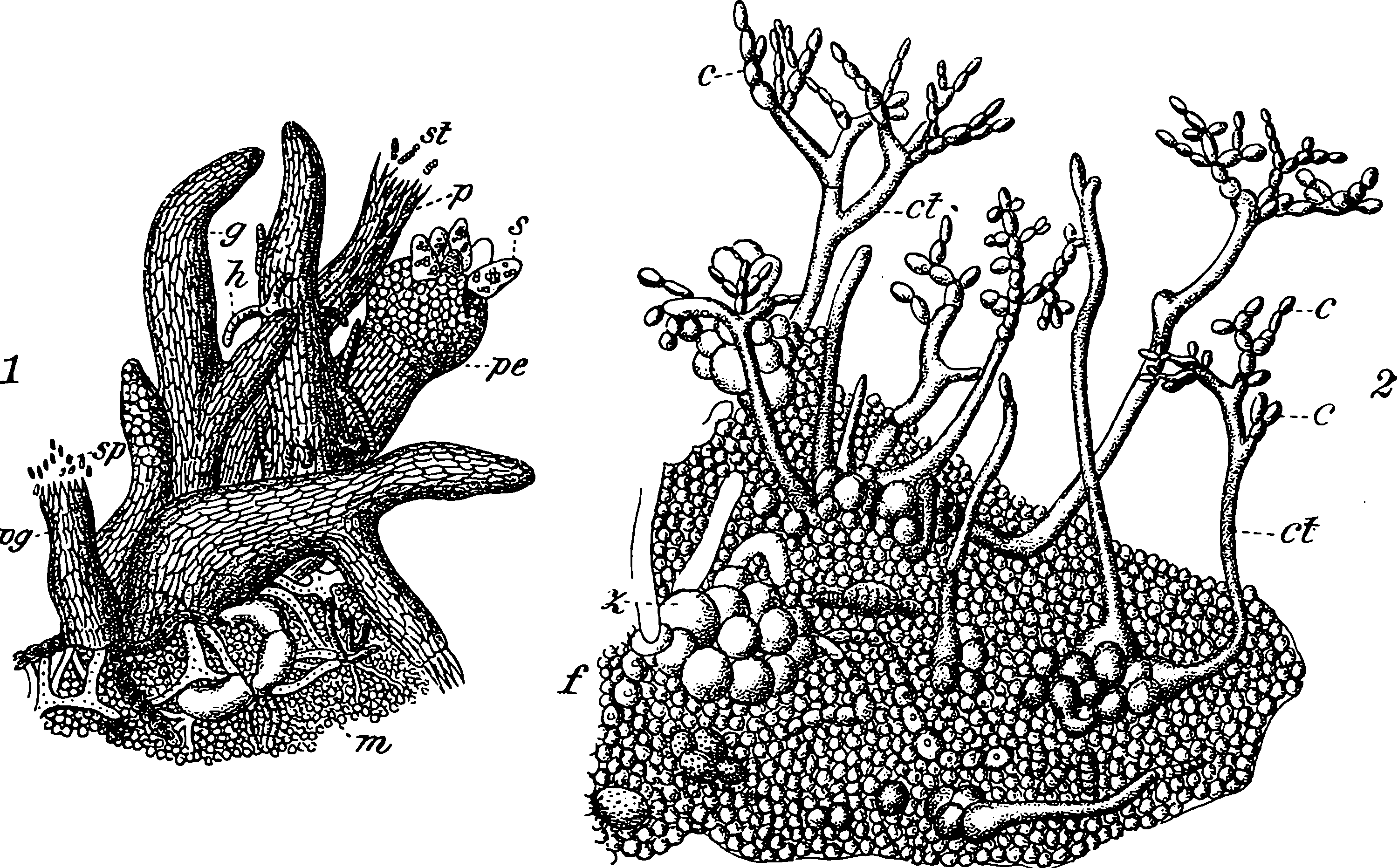# Infinite width limits of neural networksLarge-width limits of neural nets. An interesting way of considering overparameterization.

## Neural Network Gaussian Process

For now: See Neural network Gaussian process on Wikipedia.

The field that sprang from the insight that in the infinite limit, random neural nets with Gaussian weights and appropriate scaling asymptotically approach certain special Gaussian processes, and there are useful conclusions we can draw from that.

More generally we might consider correlated and/or non-Gaussian weights, and deep networks. Unless otherwise stated though, I am thinking about i.i.d. Gaussian weights, and a single hidden layer.

In this single-hidden-layer case we get tractable covariance structure. See NN kernels.For some reason this evokes multi-layer wide NNs for me

## Neural Network Tangent Kernel

NTK? See Neural Tangent Kernel.

## Implicit regularization

Here’s one interesting perspective on wide nets which looks rather like the NTK model, but is it? To read.

• The effective capacity of neural networks is large enough for a brute-force memorization of the entire data set.

• Even optimization on random labels remains easy. In fact, training time increases only by a small constant factor compared with training on the true labels.

• Randomizing labels is solely a data transformation, leaving all other properties of the learning problem unchanged.

[…] Explicit regularization may improve generalization performance, but is neither necessary nor by itself sufficient for controlling generalization error. […] Appealing to linear models, we analyze how SGD acts as an implicit regularizer.

## Dropout

Dropout is sometimes presumed to simulate from a certain kind of Gaussian process out of a neural net. See Dropout.

## As stochastic DEs

We can find an SDE for a given NN-style kernel if we can find Green’s functions $$\sigma^2_\varepsilon \langle G_\cdot(\mathbf{x}_p), G_\cdot(\mathbf{x}_q)\rangle = \mathbb{E} \big[ \psi\big(Z_p\big) \psi\big(Z_q \big) \big].$$ Russell Tsuchida observes: if you set $$G_\mathbf{s}(\mathbf{x}_p) = \psi(\mathbf{s}^\top \mathbf{x}_p) \sqrt{\phi(\mathbf{s})}$$, where $$\phi$$ is the pdf of an independent standard multivariate normal vector is a solution.

### No comments yet. Why not leave one?

GitHub-flavored Markdown & a sane subset of HTML is supported.